Home MonkeyNotes Printable Notes Digital Library Study Guides Study Smart Parents Tips College Planning Test Prep Fun Zone Help / FAQ How to Cite New Title Request

Example 43

A man of height 180 cm is moving away form a lamp-post at the rate of 1.2/sec. If the height of the lamp-post is 4.5 m. Find the rate at which the shadow is lengthening by the rate at which its tip is moving away from the lamp-post.?

Solution :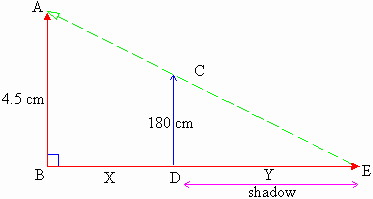AB = lamp-post,

AB = 4.5 cm

CD = Man.

CD = 180 cm =1.8 m

and distance of the tip E of the shadow from the pole = BE

Let BD = X and DE = Y

then BE = X + YYour browser does not support the IFRAME tag.

Now D ABE ~ D CDE ... (By AA test for similar triangles)

\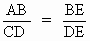\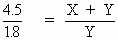\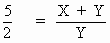\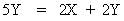\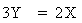\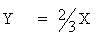Differentiating w. r. to  t .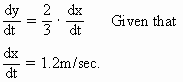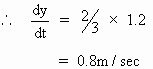\ The shadow is lengthening at the rate 0.8 m / sec.

Also BE = X + Y

\ Differentiating w. r. to  t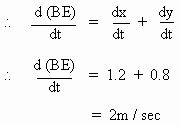\ The rate at which the tip of the shadow is moving away from the lamp-post is 2 m/sec.

Index

5.1 Tangent And Normal Lines
5.2 Angle Between Two Curves
5.3 Interpretation Of The Sign Of The Derivative
5.4 Locality Increasing Or Decreasing Functions 5.5 Critical Points
5.6 Turning Points
5.7 Extreme Value Theorem
5.8 The Mean-value Theorem
5.9 First Derivative Test For Local Extrema
5.10 Second Derivative Test For Local Extrema
5.11 Stationary Points
5.12 Concavity And Points Of Inflection
5.13 Rate Measure (distance, Velocity And Acceleration)
5.14 Related Rates
5.15 Differentials : Errors And Approximation